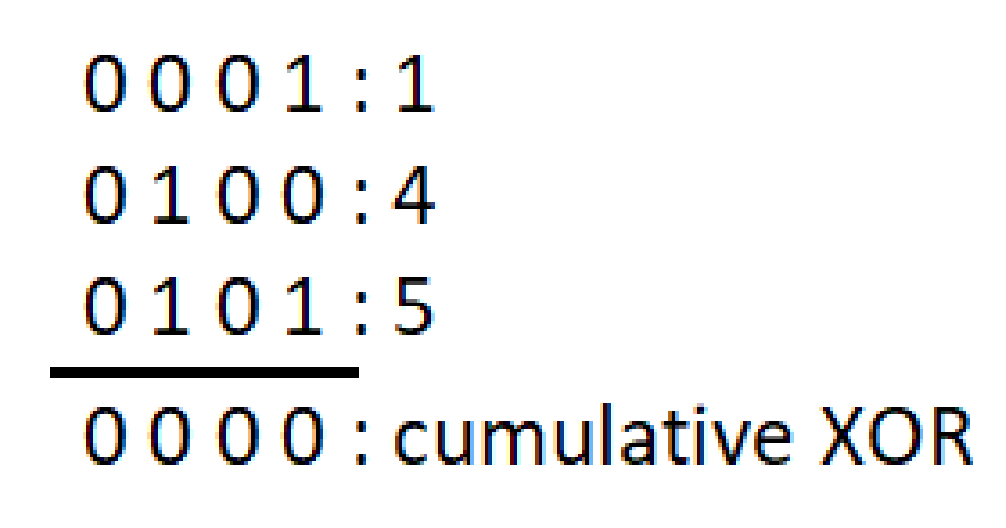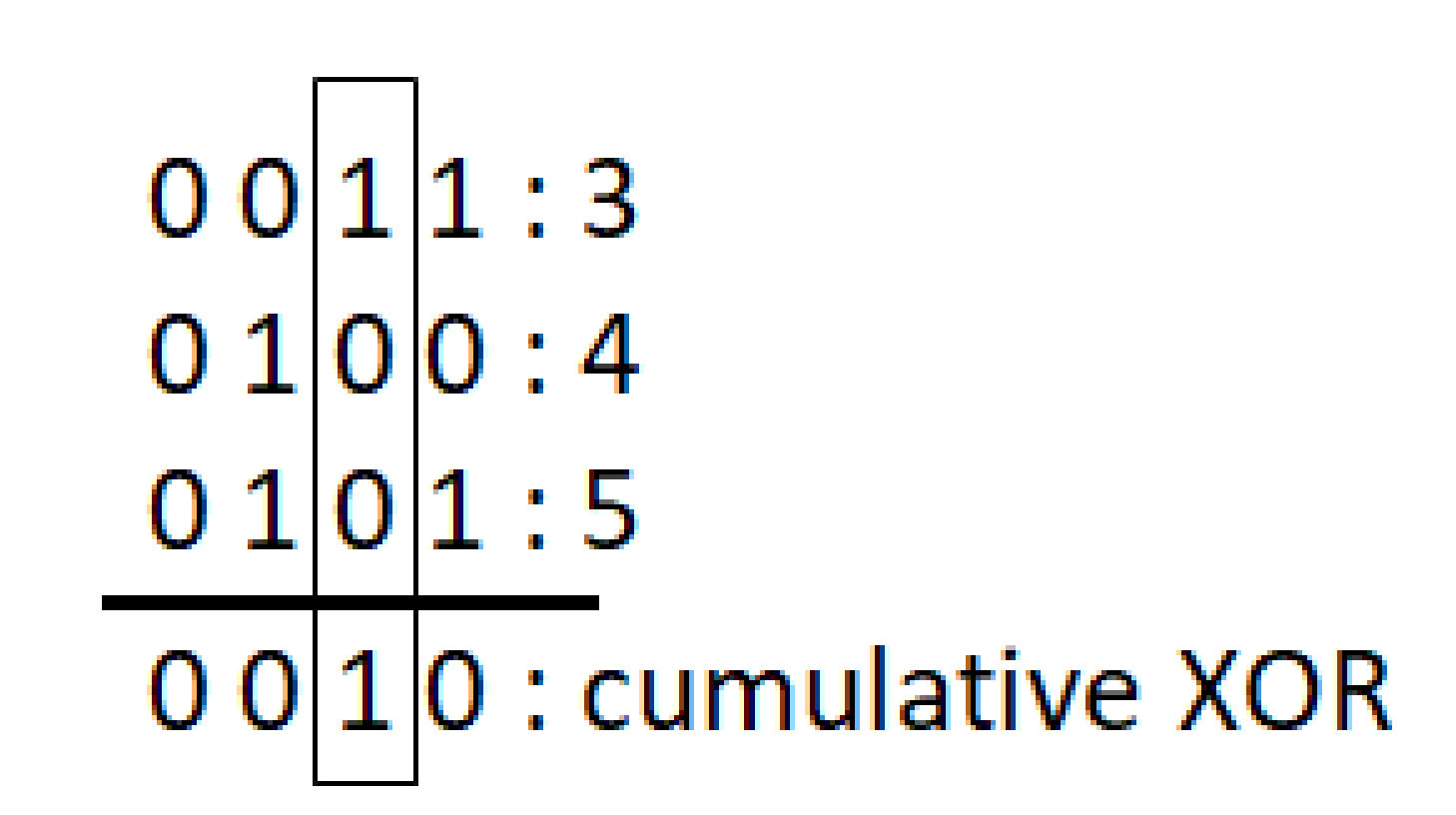New update is available. Click here to update.
Last Updated: Sep 28, 2023

# Game of NimRhythm Jain
0 upvote

## Introduction

In this article, we are going to discuss the problem “Game of Nim.” Game of Nim is a well-known mathematics game in which two players alternately take items from piles.The game is won by the one who picks the last. For any number of beginning piles and objects, the Game of Nim can be solved mathematically.

Game of Nim is a practical example of game theory and combinatorial games.

To understand this problem more deeply, let’s dive into the problem statement.

For our clear understanding, let the “object” be “coins.”

## Problem Statement

There are “n” numbers of piles where each pile contains some amount of coins. There are two players, A and B. Two players will play alternatively and optimally. In each turn, a player can choose any pile and can remove any number of coins from the pile. Players must choose at least one coin for the game to proceed. The player who picks last wins the game, or, in other words, the player who has no moves left loses the game.

Our task is to find the winner even before the game starts if both the players play optimally.

## Example

Consider two players, A and B, and initially, there are three piles of coins having 3,

4, and 5 coins, respectively.

We assume A starts the game, and the moves A and B take are as follows:

● A takes 2 coins from the first pile. The coins left in 3 piles are 1,4, and 5.

● B takes 3 coins from the third pile. The coins left in 3 piles are 1,4, and 2.

● A takes 1 coin from the second pile. The coins left are 1,3, and 2.

● B takes 1 coin from the second pile. The coins left are 1,2, and 2.

● A removes all the coins from the first pile. The coins left are 0,2 and 2.

● B removes 1 coin from the second pile. The coins left are 0,1, and 2.

● A removes 1 coin from the third pile. The coins left are 0,1, and 1.

● B removes all the coins from the second pile. The coins left are 0,0 and 1.

● Lastly, A removes the last coin from pile 3 and wins the game.

In this game, A won the match, but A also made the first move. If B started the game, then B

must have won. So the final answer depends on who started the game

But will it always be like this, that the player who started the game will always win? Consider

another scenario where there are again 3 piles but having 1,4 and 5 coins respectively.

Let A make the first move. The game goes as follows:

● A takes 3 coins from the third pile. The coins left in 3 piles are 1,4, and 2.

● B takes 1 coin from the second pile. The coins left in 3 piles are 1,3, and 2.

● A takes 1 coin from the second pile. The coins left are 1,2, and 2.

● B removes all the coins from the first pile. The coins left are 0,2 and 2.

● A removes 1 coin from the second pile. The coins left are 0,1, and 2.

● B removes 1 coin from the third pile. The coins left are 0,1, and 1.

● A removes 1 coin from the second pile. The coins left are 0,0 and 1.

● B removes all the coins from the third pile and wins the game. The coins left are

0,0 and 0.

So, the second factor on which the winner depends is the initial stage of the piles.

How can we predict the winner before even playing the game?

## Approaching Solution

We have to find the winner such that both Player A and Player B play optimally.

To represent the pile and number of coins in the ith pile, suppose we have an array called “piles” of length n such that there are n piles and piles[i] is the number of coins in an ith pile.

From the above example, it is pretty evident that the winner of the game depends on the initial configuration of the piles and the turn of players.

Let us define a Term called Nim-Sum.

## Nim-Sum

Nim-Sum is the cumulative XOR value of the number of coins in each pile at any stage throughout the game.

Suppose both players play optimally, i.e., without making any mistakes. In that case, the player who makes the first move is guaranteed to win if the nim-sum of the initial stage of piles is non-zero.

### Proof

• Assume that the XOR sum of 'n' numbers is zero. In that instance, a single number decrease will not be enough to make the XOR sum zero.

Example: Consider 3 piles having 1, 4, and 5 coins having cumulative XOR 0.Suppose you remove any number of coins from a single pile. In that case, you will have to toggle a single bit or multiple bits from the binary representation of that pile. It will always result in a non-zero cumulative XOR.

• If the XOR sum of 'n' numbers is not zero, then there is at least one method by which the XOR sum can be reduced to zero.

Example: Consider 3 piles having 3, 4, and 5 coins having non-zero cumulative XOR.You can always remove some x number of coins from some pile to make a cumulative XOR of 0. In this example, you can remove 2 coins from the first pile to make the second set bit from right 0. Then there will be an even number of set bits, and the cumulative XOR will be 0.

Now, for the Nim Game, two cases can exist:

Case 1: Initially, nim sum is zero, and A makes the first move.

According to the optimal strategy, A must now pick at least one coin, causing the Nim-Sum to be non-zero. As explained above, B can always make the nim-sum zero because the nim sum is already non-zero in B's turn. This will continue, with A making the nim-sum non-zero and B making the nim-sum zero. Finally, B will remove all of the coins from some piles, resulting in a binary representation with zeros in all positions across all piles, causing A to lose the game.

Case 2: Initially, nim sum is non-zero, and A makes the first move

According to the best strategy, A should now make the Nim-Sum zero (possible as the initial Nim sum is non-zero, as mentioned above). Because the nim sum is already zero, whatever number B chooses will make the nim sum non-zero. A can choose a number to make the nim sum zero again. This will continue as long as goods are available in each pile, with A picking the final item.

## Code

Output:

### Complexity Analysis

#### Time Complexity: O(N)

Where N is the number of piles.

Since we are iterating over the array, it is linear time-based.

#### Space Complexity: O(1)

1. What is Nim Sum?

Nim Sum is the cumulative XOR value of the number of coins in each pile at any game point.

2. What are the two factors on which the winner of the game depends?

The winner of the game depends on :

• The player who starts first.
• The initial configuration of the piles/heaps.

## Key Takeaways

Game theory helps you to predict the output of a game without actually playing the game for games based on combinatorics. Game theory is quite considerable in mathematics, and with some mathematical theorems, we can solve games like ‘Game of Nim.’

What's nice is that.’ Game theory actually tells us how to construct the winning move (if one exists) for any given board state.

Check out this problem - Two Sum Problem